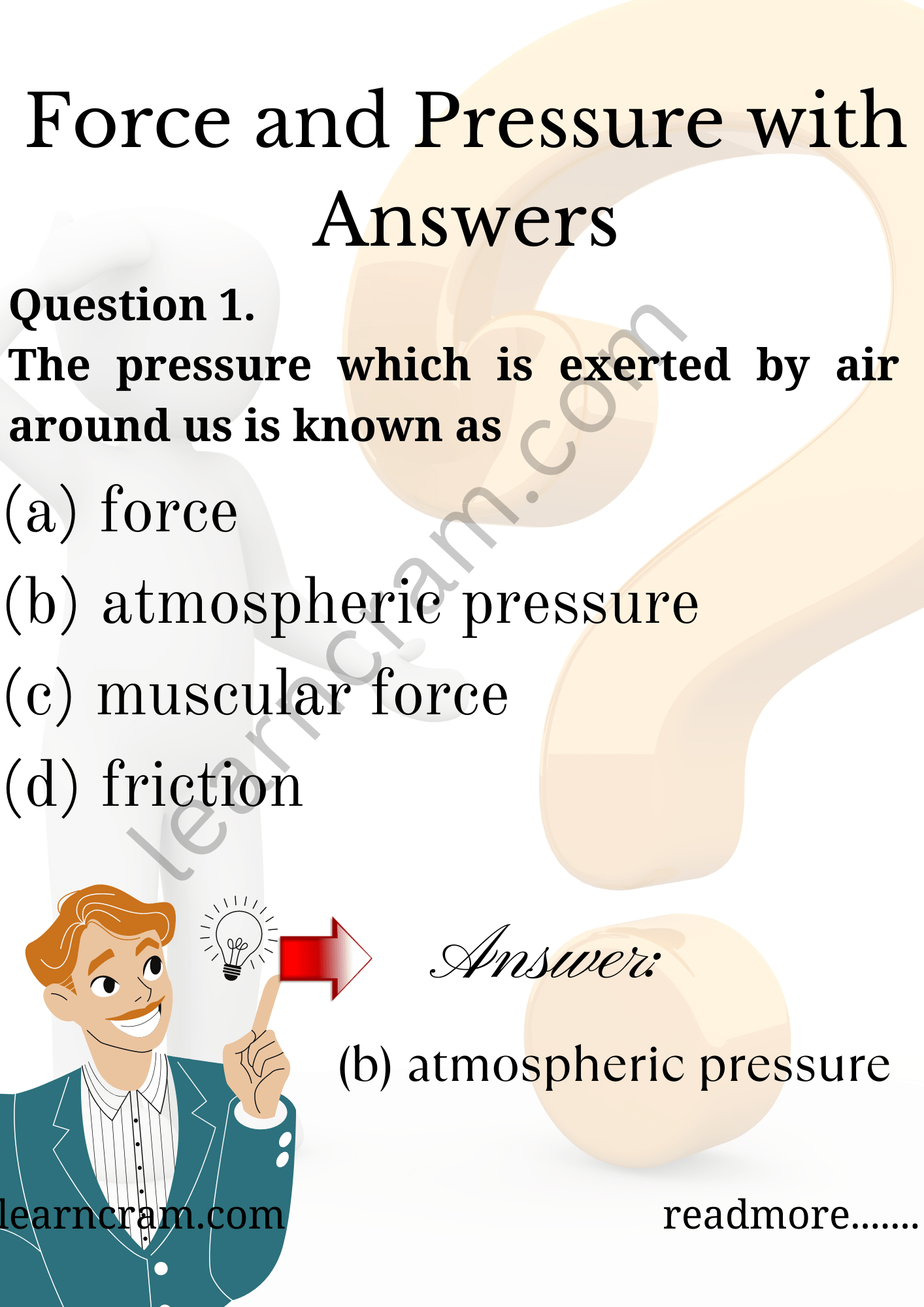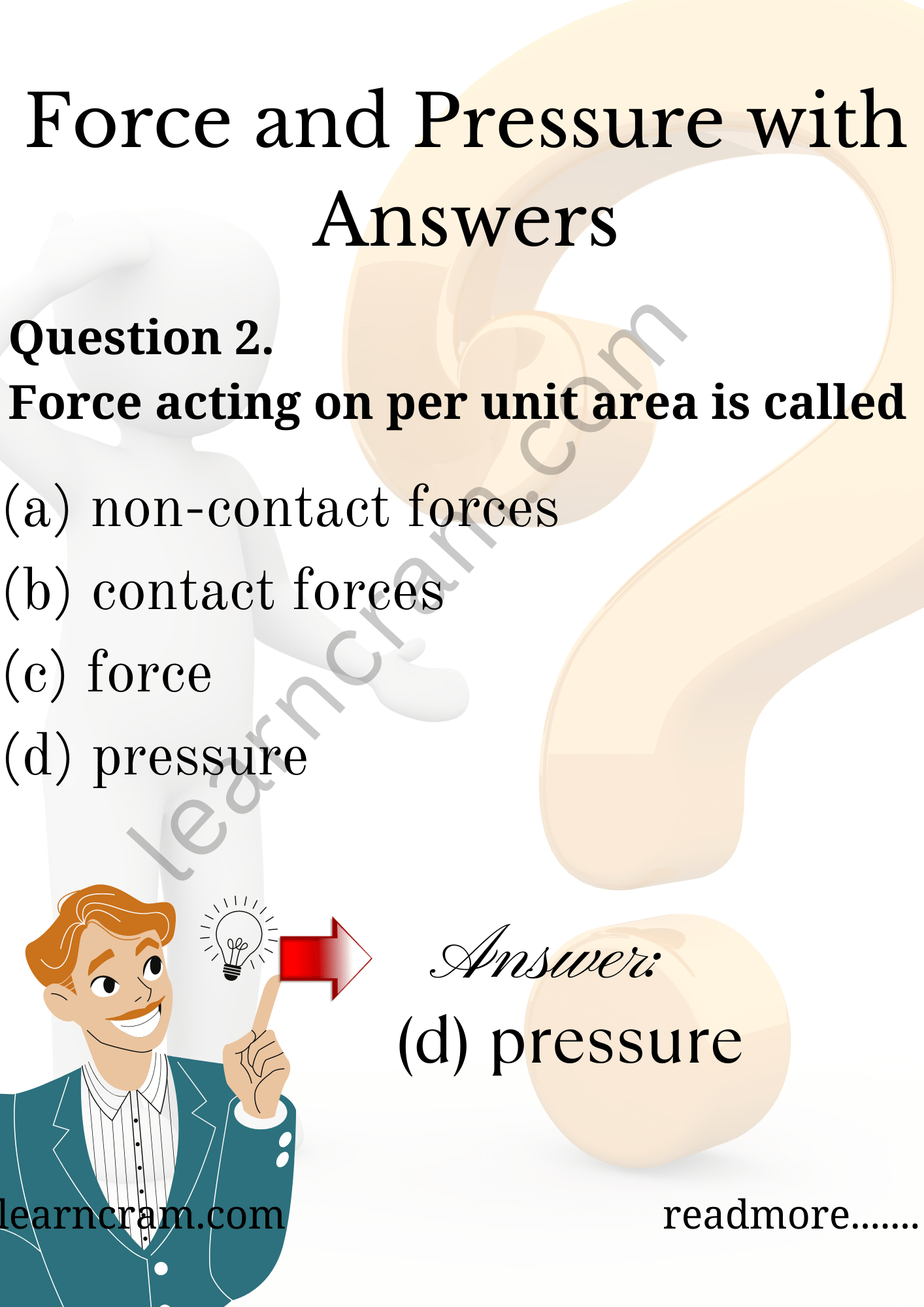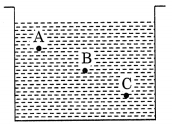# MCQ Questions for Class 8 Science Chapter 11 Force and Pressure with Answers

We have compiled the NCERT MCQ Questions for Class 8 Science Chapter 11 Force and Pressure with Answers Pdf free download covering the entire syllabus. Practice MCQ Questions for Class 8 Science with Answers on a daily basis and score well in exams. Refer to the Force and Pressure Class 8 MCQs Questions with Answers here along with a detailed explanation.

## Force and Pressure Class 8 MCQs Questions with Answers

Choose the correct option.

Question 1.
The pressure which is exerted by air around us is known as
(a) force
(b) atmospheric pressure
(c) muscular force
(d) frictionQuestion 2.
Force acting on per unit area is called
(a) non-contact forces
(b) contact forces
(c) force
(d) pressureQuestion 3.
A exerted by an object on another is a force.
(a) Push or pull
(b) Contact or non-contact force
(c) Pressure
(d) Magnitude

Question 4.
The force exerted by the earth to pull the object towards itself is called
(a) electrostatic force
(b) gravitational force
(c) muscular force
(d) contact force

Question 5.
Muscular force is also called __________ force.
(a) non-contact
(b) contact
(c) gravitational
(d) magnetic

Question 6.
The force exerted by a charged body on another charged or uncharged body is called
(a) gravitational force
(b) electrostatic force
(c) non-contact force
(d) contact force

Question 7.
Force changes the
(a) motion of body
(b) speed of body
(c) shape of body
(d) all of these

Question 8.
The force exerted by our muscle is called
(a) electrostatic force
(b) muscular force
(c) gravitational force
(d) non-contact force

Question 9.
1 kilogram weight is equal to
(a) 98 N
(b) 9.8 N
(c) 0.98 N
(d) 0.098 N

Question 10.
A spring balance is used for measuring
(a) mass
(b) weight
(c) pressure
(d) speed

Question 11.
Two boys A and B are applying force on a block. If the block moves towards the boy A, which one of the following statements is correct?
(a) Magnitude of force applied by A is greater than that of B.
(b) Magnitude of force applied by A is smaller than that of B.
(c) Net force on the block is towards B.
(d) Magnitude of force applied by A is equal to that of B.

Answer: (b) Magnitude of force applied by A is smaller than that of B.

Question 12.
When two forées act in opposite directions, then net force acting is the
(a) sum of two forces
(b) difference between two forces
(c) both of these
(d) none of these

Answer: (b) difference between two forces

Question 13.
The strength of force is expressed by its
(a) weight
(b) mass
(c) magnitude
(d) longitudinal force

Question 14.
Leaves fall down on the ground due to
(a) electrostatic force
(b) magnetic force
(c) gravitational force
(d) muscular force

Question 15.
State of motion is described by
(a) Position of rest
(b) Position of motion
(c) Both by the state of rest or motion
(d) None of these

Answer: (c) Both by the state of rest or motion

Question 16.
When the hammer strikes the gong of an electric bell, which of the following force is responsible for the movement of hammer?
(a) Gravitational force alone
(b) Magnetic force alone
(c) Electrostatic force alone
(d) Frictional force alone

Question 17.
During dry weather, while combing hair, sometimes we experience hair flying apart. The force responsible for this is
(a) force of gravity
(b) force of friction
(c) electrostatic force
(d) magnetic force

Question 18.
A container is filled with water as shown in the given figure. Which of the following statements is correct about pressure of water?(a) Pressure at A > Pressure at B > Pressure at C
(b) Pressure at A = Pressure at B = Pressure at C
(c) Pressure at A < Pressure at B > Pressure at C
(d) Pressure at A < Pressure at B < Pressure at C

Answer: (d) Pressure at A < Pressure at B < Pressure at C

Question 19.
Two objects repel each other. This repulsion could be due to
(a) frictional force only
(b) electrostatic force only
(c) magnetic force only
(d) either a magnetic or an electrostatic force

Answer: (d) either a magnetic or an electrostatic force

Question 20.
Which one of the following forces is a contact force?
(a) Force of gravity
(b) Magnetic force
(c) Force of friction
(d) Electrostatic force

Question 21.
A brick is kept in three different ways on a table as shown in given figure. The pressure exerted by the brick on the table will be(a) maximum in position A
(c) maximum in position B
(b) maximum in position C
(d) equal in all cases

Answer: (a) maximum in position A

Question 22.
Which force is being applied by a vendor when he is pushing the pushcart?
(a) Muscular force
(b) Magnetic force
(c) Frictional force
(d) Electrostatic force

Question 23.
Which force always opposes motion?
(a) Magnetic force
(b) Electrostatic force
(c) Frictional force
(d) Muscular force

Question 24.
Which of the following exerts gravitational force?
(a) Earth
(b) Venus
(c) Saturn
(d) All of the above

Answer: (d) All of the above

Question 25.
Which force causes a charged balloon to attract another balloon?
(a) Muscular force
(b) Electrostatic force
(c) Magnetic force
(d) Gravitational force

Question 26.
What is the SI unit of force?
(a) Newton
(b) Galileo
(c) Einstein
(d) Pascal

Question 27.
As we go to the higher altitudes, the atmospheric pressure
(a) increases
(b) decreases
(c) remains the same
(d) cannot say

Question 28.
The pressure of the water at the bottom of the pond is than at the surface.
(a) lower
(b) higher
(c) same
(d) either lower or higher

Question 29.
A body is said to be under balanced forces when the resultant of all the forces applied is
(a) one
(b) zero
(c) infinite
(d) none of these

Question 30.
Opening a door is an example of a
(a) contact force
(b) non-contact force
(c) muscular force
(d) both (a) and (c)

Answer: (d) both (a) and (c)

Question 31.
What is the cause of change in the state of motion of an object?
(a) Pressure
(b) Atmospheric pressure
(c) Friction
(d) Force

Fill in the Blanks

Question 1.
We ………………. the bucket to take out water from well.

Question 2.
The strength of a force is usually expressed by its ……………….

Question 3.
………………. force is the force resulting due to the action of muscles.

Question 4.
The envelop of air all around us is called ……………….

Question 5.
To open a door we or ………………. the door.

Question 6.
Force of friction is an example of ………………. force.

Question 7.
Force ………………. is called pressure.

Question 8.
Force has ………………. as well as direction.

Question 9.
Fruits fall ………………. due to the force of gravity.

Question 10.
The body at rest is called ………………. body.

Question 11.
………………. and ………………. forces are the two kinds of forces.

Question 12.
Force exerted by magnet is called ………………. force.

Question 13.
We apply force on ………………. while walking.

Question 14.
Force is ………………. to pressure.

Question 15.
The ………………. is measured by an instrument called barometer.

Question 16.
Force acting on a body can change its ……………………. and state of motion.

Question 17.
The wear and tear in the machine parts is due to …………………….

Question 18.
A force arises due to ……………………. between two objects.

Question 19.
The pressure exerted by a liquid ……………………. with depth.

Question 20.
Like poles of a magnet ……………………. each other.

Question 21.
A drinking straw works on the principle of ……………………. pressure.

True or False

Question 1.
Force applied on an object in opposite directions add to one another.

Question 2.
Pressure does not depend on area of contact.

Question 3.
A force can change the state of motion of an object.

Question 4.
Force cannot change the speed of an object.

Question 5.
Atmospheric pressure is less at higher altitudes.

Question 6.
Force of gravity is a contact force.

Question 7.
Muscular force is also known as contact force.

Question 8.
Pascal is the unit of force.

Question 9.
To move an object faster it has to be pushed or pulled repeatedly.

Question 10.
Magnitude is the strength of force.

Question 11.
Force can change the shape of an object.

Question 12.
At least two objects must interact for a force to come into play.

Question 13.
Magnetic force is a non-contact force.

Question 14.
An apple from a tree falls on the ground due to the force of gravity.

Question 15.
Newton is the unit of force.

Question 16.
Fluids exert pressure on the walls of their container.

Question 17.
A piece of rubber regains its shape when force is removed.

Question 18.
Cutting and piercing tools have blunt edges.

Question 19.
Camel cannot walk on sand due to its broad feet.

Question 20.
Non-contact force can act from a distance.

Question 21.
The syringe works on the principle of atmospheric pressure.

Question 22.
People in plains suffer from nose bleeding.

Question 23.
Electrostatic force is a contact force.

Question 24.
Air resistance opposes motion of objects through the air.

Question 25.
The SI unit of pressure is newton.

Match the following

 Column I Column II 1. Pressure (a) Fluid pressure 2. Barometer (b) Unit of pressure 3. Newton (c) Non-contact force 4. Manometer (d) Force/Area 5. Pascal (e) Unit of force 6. Magnetic force (f) Net force acting on a body 7. 1 kilogram (g) Atmospheric pressure 8. Resultant force (h) 9.8 N 9. Frictional force (i) Down the column 10. Pressure increases (j) Contact force